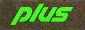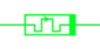# Compute Pi

7 posts / 0 newOffline
Last seen: 5 months 6 days ago
Joined: Sep 19 2022 - 15:54
Posts: 14
Compute Pi

Can the Apple 2 Compute pi? If so what software?Offline
Last seen: 3 days 2 hours ago
Joined: Dec 19 2003 - 17:34
Posts: 1737
Yes, it can be done in BASIC.

Yes, it can be done in BASIC. See page 15 of this journal from 1978: http://archive.6502.org/publications/micro/micro_06_aug_1978.pdfOffline
Last seen: 1 month 2 weeks ago
Joined: Aug 18 2005 - 16:26
Posts: 408
?4*ATN(1)

]`PI=3.1415926535`

]`PRINT PI`

3.14159266

How much precision were you looking for?

I am particular to tau: τ which is 2 * PI

]`TAU = 8 * ATN(1)`

]`PRINT TAU`

6.28318531Offline
Last seen: 1 day 11 hours ago
Joined: Aug 9 2022 - 00:48
Posts: 535
I don't think you get the

I don't think you get the point. The question was about computing PI to a very large number of decimals, not just 8.Online
Last seen: 30 min 57 sec ago
Joined: Oct 15 2012 - 08:59
Posts: 795
Clever way to calculate Pi

I found this to be a clever way to calculate Pi.  Not sure who came up with it, but it would be easy to write a basic program to do this.

Take 4 and subtract "4 divided by 3".

Then add "4 divided by 5".

Then subtract "4 divided by 7".

Continue alternating between adding and subtracting fractions with a numerator of 4 and a denominator of each subsequent odd number.

The more times you do this, the closer you will get to pi.

It would be nice to compare the calculation time to (say) 20 digits or so using Applesoft Basic vs. a Binary run-able calculation.

Offline
Last seen: 11 hours 24 sec ago
Joined: Feb 27 2021 - 18:59
Posts: 320
Taylor

It's an application of the Taylor series. See https://math.hmc.edu/funfacts/taylor-made-pi/

Offline
Last seen: 1 day 21 hours ago
Joined: Jun 12 2022 - 23:35
Posts: 94
That's the Leibniz series:Pi

That's the Leibniz series:

Pi/4 = 1 - 1/3 + 1/5 - 1/7 ....

It is based on the Taylor series for the arctangent function

ATAN(X) = X - X/3 + X/5 - X/7 ...

knowing that ATN 1 = pi/4

unfortunately it converges logarithmically.  Basically you have to double the number of terms you need for each additional digit of accuracy.  Literally millions of terms are needed for 6 or 7 digits of accuracy.

The most efficient series I believe is the Bailey-Borwein-Plouffe formula and Bellard's formula

https://en.wikipedia.org/wiki/Bailey%E2%80%93Borwein%E2%80%93Plouffe_formula

https://en.wikipedia.org/wiki/Bellard%27s_formula

It can directly generate binary digits of pi.  It is used in all of the big pi computation projects.